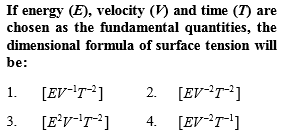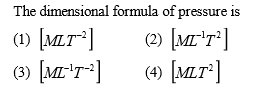You’ve reached the end of your free Videos limit.
#1 | Basic Concepts & Examples
(Physics) > Units and Measurement
Related Practice Questions :Complete Question Bank + Test Series
Complete Question Bank

Difficulty Level:Complete Question Bank + Test Series
Complete Question Bank

Difficulty Level:

Light year is a unit of

(1) Time

(2) Mass

(3) Distance

(4) Energy

Complete Question Bank + Test Series
Complete Question Bank

Difficulty Level:

Which of the following is not represented in correct unit

(1) $\frac{\text{Stress}}{\text{Strain}}=N\text{​}/\text{​}{m}^{2}$

(2) Surface tension = $N\text{​}/\text{​}m$

(3) Energy $=kg\text{​-​}m\text{​}/\text{​}\mathrm{sec}$

(4) Pressure $=N\text{​}/\text{​}{m}^{2}$

Complete Question Bank + Test Series
Complete Question Bank

Difficulty Level:

The unit of power is

(1) Joule

(2) Joule per second only

(3) Joule per second and watt both

(4) Only watt